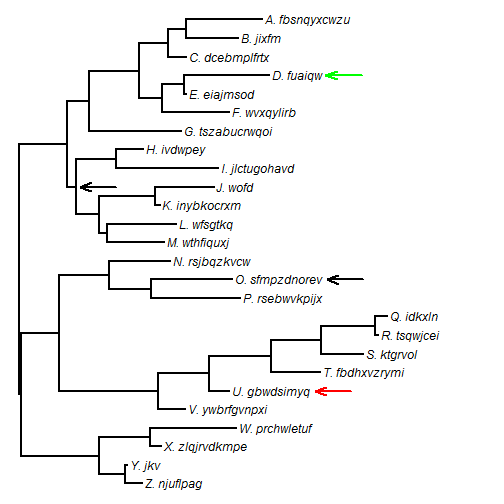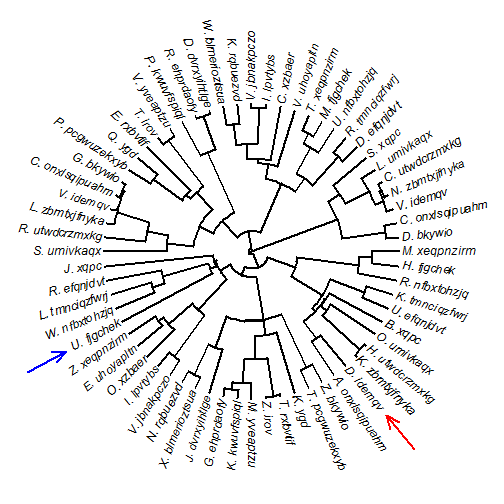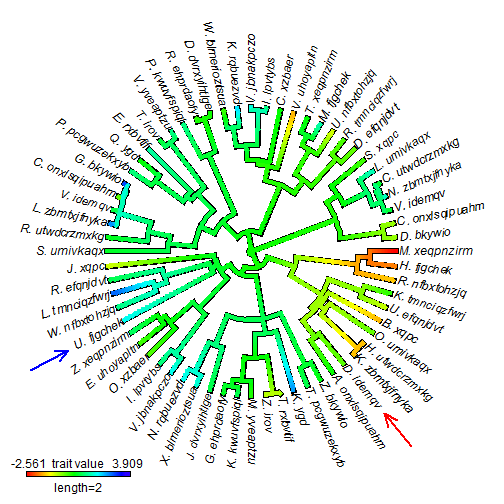## Friday, December 26, 2014

### Wrapper function to optimize the λ tree transformation for a discrete trait

Today I responded to an R-sig-phylo query by posting some code to optimize Pagel's λ tree transformation for a discrete character evolving by a continuous-time Markov chain. I did this by writing a very simple wrapper around ape's `ace` function for ancestral character estimation, which also fits this model:

``````## here's the wrapper function
fitLambda<-function(tree,x,model="ER"){
lik<-function(lambda,tree,x,model)
logLik(ace(x,rescale(tree,model="lambda",lambda),
type="discrete",model=model))
obj<-optimize(lik,c(0,1),tree=tree,x=x,model=model,maximum=TRUE)
fit<-ace(x,rescale(tree,model="lambda",lambda=obj\$maximum),
type="discrete",model=model)
I<-fit\$index.matrix
fitted.Q=matrix(fit\$rates[I],dim(I),dim(I),
dimnames=list(dimnames(fit\$lik.anc)[],
dimnames(fit\$lik.anc)[]))
diag(fitted.Q)<--rowSums(fitted.Q,na.rm=TRUE)
list(Q=fitted.Q,lambda=obj\$maximum,logLik=logLik(fit))
}
library(geiger)
library(phytools)
## simulate some data to test it
tree<-pbtree(n=200,scale=1)
Q<-matrix(c(-1,1,1,-1),2,2)
rownames(Q)<-colnames(Q)<-letters[1:2]
x<-sim.history(rescale(tree,model="lambda",lambda=0.7),Q)\$states
``````
``````## Done simulation(s).
``````
``````fitLambda(tree,x)
``````
``````## \$Q
##         a       b
## a -0.7995  0.7995
## b  0.7995 -0.7995
##
## \$lambda
##  0.5154
##
## \$logLik
##  -128.6
``````

Note that the same model can also be fit using geiger's `fitDiscrete` function, but the poster reported some issues with convergence which made me want to post the second way. Here is `fitDiscrete`

``````fitDiscrete(tree,x,transform="lambda")
``````
``````## DLSODA-  Warning..Internal T (=R1) and H (=R2) are
##       such that in the machine, T + H = T on the next step
##      (H = step size). Solver will continue anyway.
## In above message, R1 = 0, R2 = 0
##
## DINTDY-  T (=R1) illegal
## In above message, R1 = 2.8036e-222
##
##       T not in interval TCUR - HU (= R1) to TCUR (=R2)
## In above message, R1 = 0, R2 = 0
##
## DINTDY-  T (=R1) illegal
## In above message, R1 = 8.66551e-221
##
##       T not in interval TCUR - HU (= R1) to TCUR (=R2)
## In above message, R1 = 0, R2 = 0
##
## DLSODA-  Trouble in DINTDY.  ITASK = I1, TOUT = R1
## In above message, I1 = 1
##
## In above message, R1 = 8.66551e-221
##
``````

Here I've excluding a whole bunch of error messages....

``````##
## In above message, R1 = 8.66551e-221
##
``````
``````## GEIGER-fitted comparative model of discrete data
##  fitted Q matrix:
##             a       b
##     a -0.7928  0.7928
##     b  0.7928 -0.7928
##
##  fitted 'lambda' model parameter:
##  lambda = 0.508644
##
##  model summary:
##  log-likelihood = -128.569446
##  AIC = 261.138891
##  AICc = 261.199805
##  free parameters = 2
##
## Convergence diagnostics:
##  optimization iterations = 100
##  failed iterations = 56
##  frequency of best fit = NA
##
##  object summary:
##  'lik' -- likelihood function
##  'bnd' -- bounds for likelihood search
##  'res' -- optimization iteration summary
##  'opt' -- maximum likelihood parameter estimates
``````

Also, fitting the λ model to discrete trait data has always struck me as a somewhat peculiar enterprise, so this is posted without any prejudice towards whether this is a good idea, a bad idea, or an average idea in the first place!

## Monday, December 15, 2014

### New (non-CRAN) version of phytools; more on new phytools function add.arrow

I just posted a new non-CRAN version of phytools (go here and pick the latest version, which can be installed from source). I'm working towards an update to phytools on CRAN; but I need to do some more debugging of phytools' new functions, such as `locate.yeti` and `locate.fossil`, as well as add some details to new documentation, before I do that.

This latest version now includes the aforementioned `locate.fossil`. It also includes `fitPagel`, a relatively simple wrapper function that implements Pagel's 1994 method to test for correlated evolution of two binary characters, as well as the brand new function `add.arrow`, which adds an arrow to a plotted radial or square phylogram.

Here's a quick demo of the last function in this list:

``````library(phytools)
packageVersion("phytools")
``````
``````##  '0.4.42'
``````
``````## simulate tree with realistic tip labels
set.seed(10)
tree<-rtree(n=26)
tree\$tip.label<-paste(LETTERS[26:1],"._",sep="",
replicate(26,paste(sample(letters,sample(3:12,1)),
collapse="")))
h<-max(nodeHeights(tree))
plotTree(tree,ftype="i")
## we can do it without supplying a tree as a function argument
## we can have an arrow point to a node
``````Of course, as described in the original post the function also works for radial (`type="fan"`) trees, e.g.:

``````tree<-pbtree(n=64)
tree\$tip.label<-paste(sample(LETTERS,64,replace=TRUE),"._",sep="",
replicate(26,paste(sample(letters,sample(3:12,1)),
collapse="")))
h<-max(nodeHeights(tree))
plotTree(tree,type="fan",ftype="i")
``````
``````## setEnv=TRUE for this type is experimental. please be patient with bugs
``````
``````add.arrow(tree,tip="D._idemqv",col="red")
``````And we can use it for plotted objects of class `"contMap"` and `"densityMap"`, e.g.:

``````x<-fastBM(tree)
obj<-contMap(tree,x,plot=FALSE)
plot(obj,type="fan",legend=2)